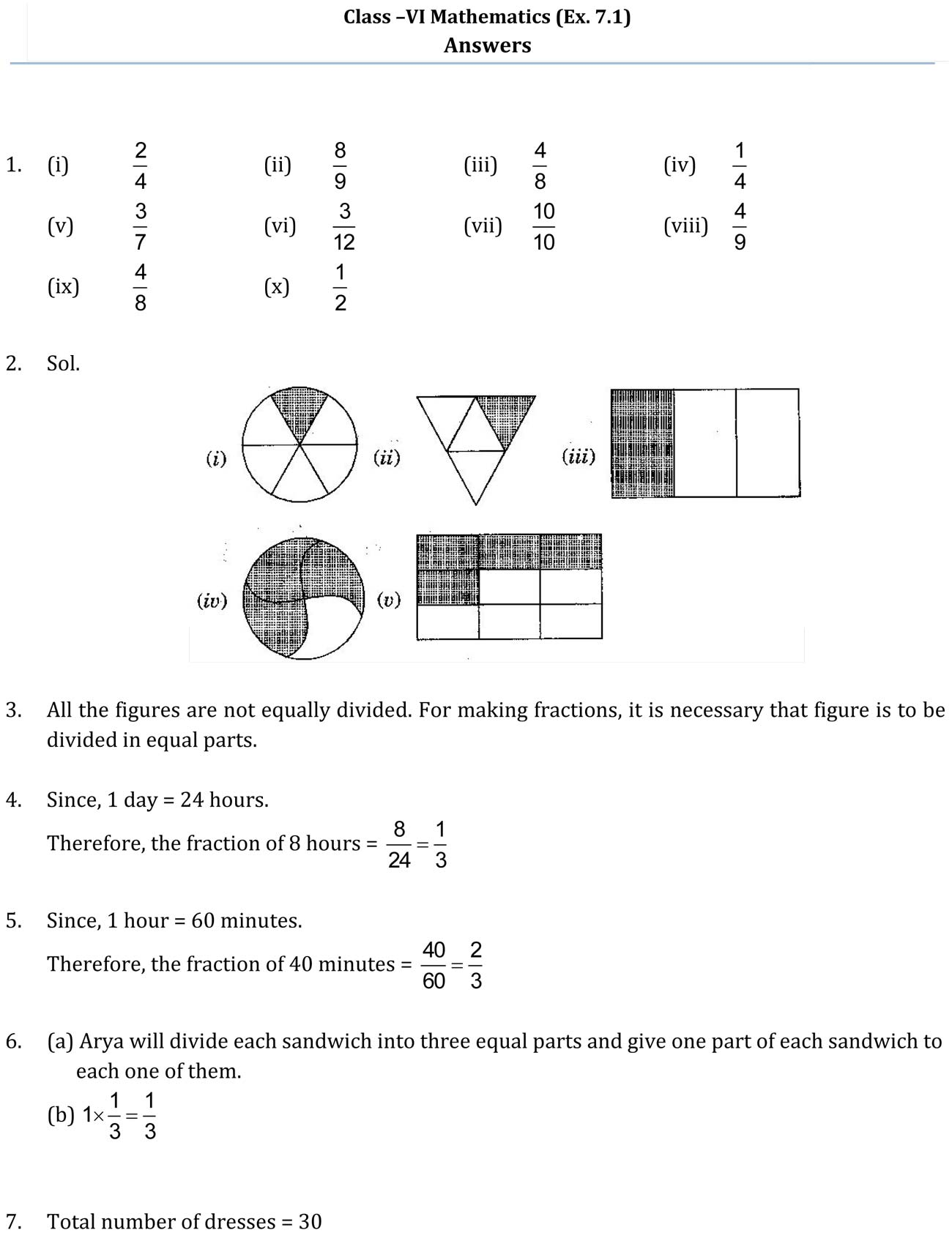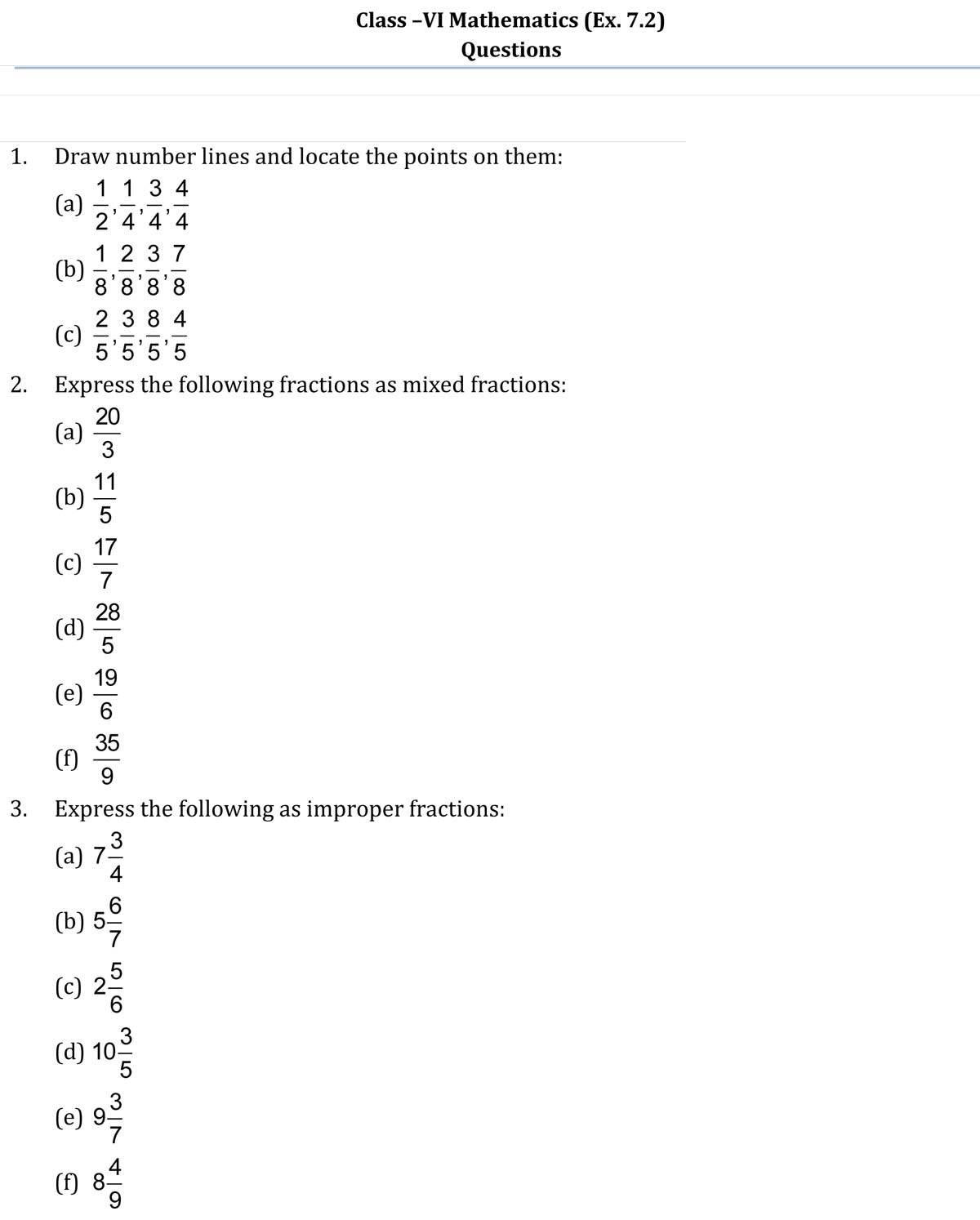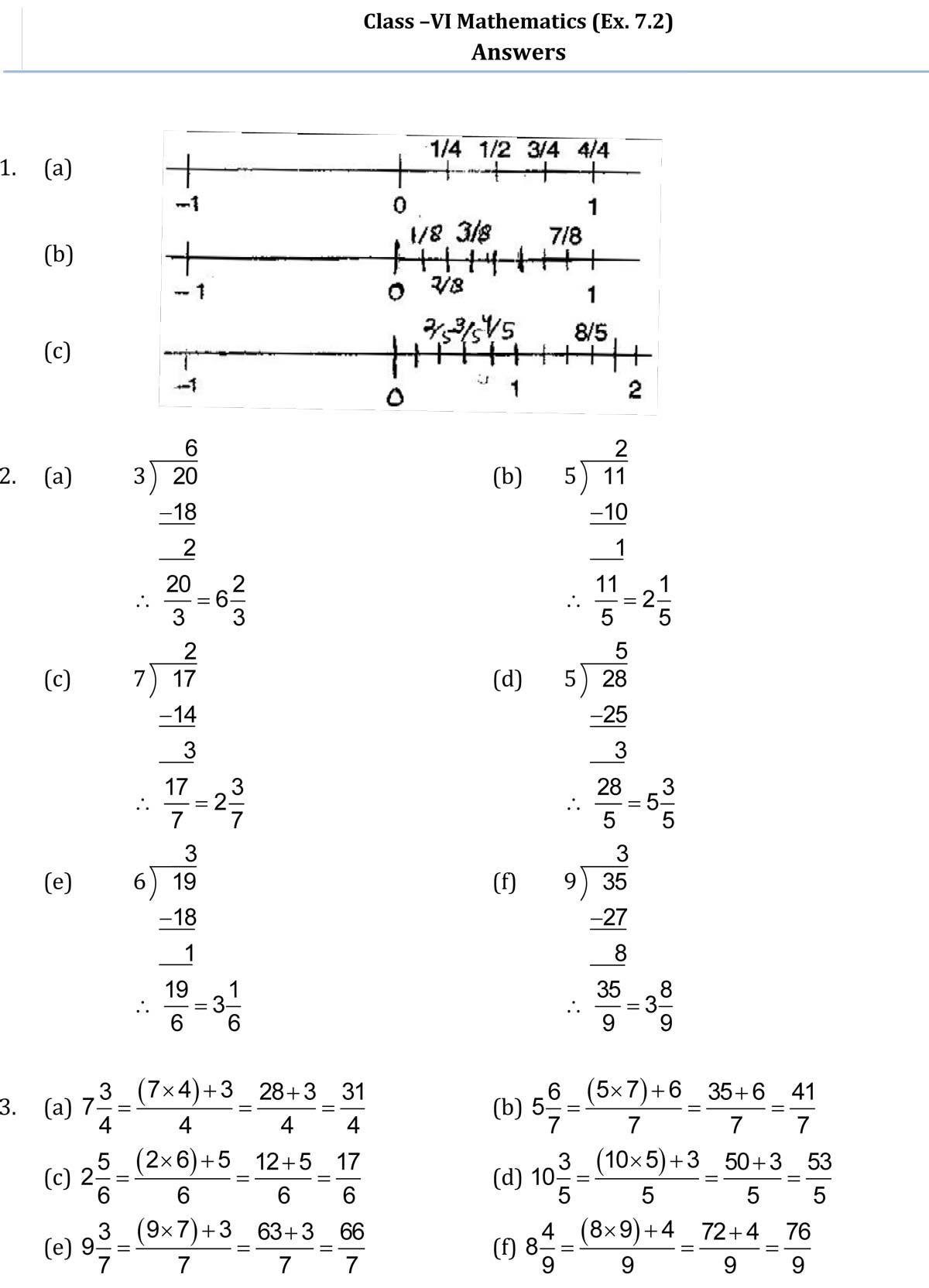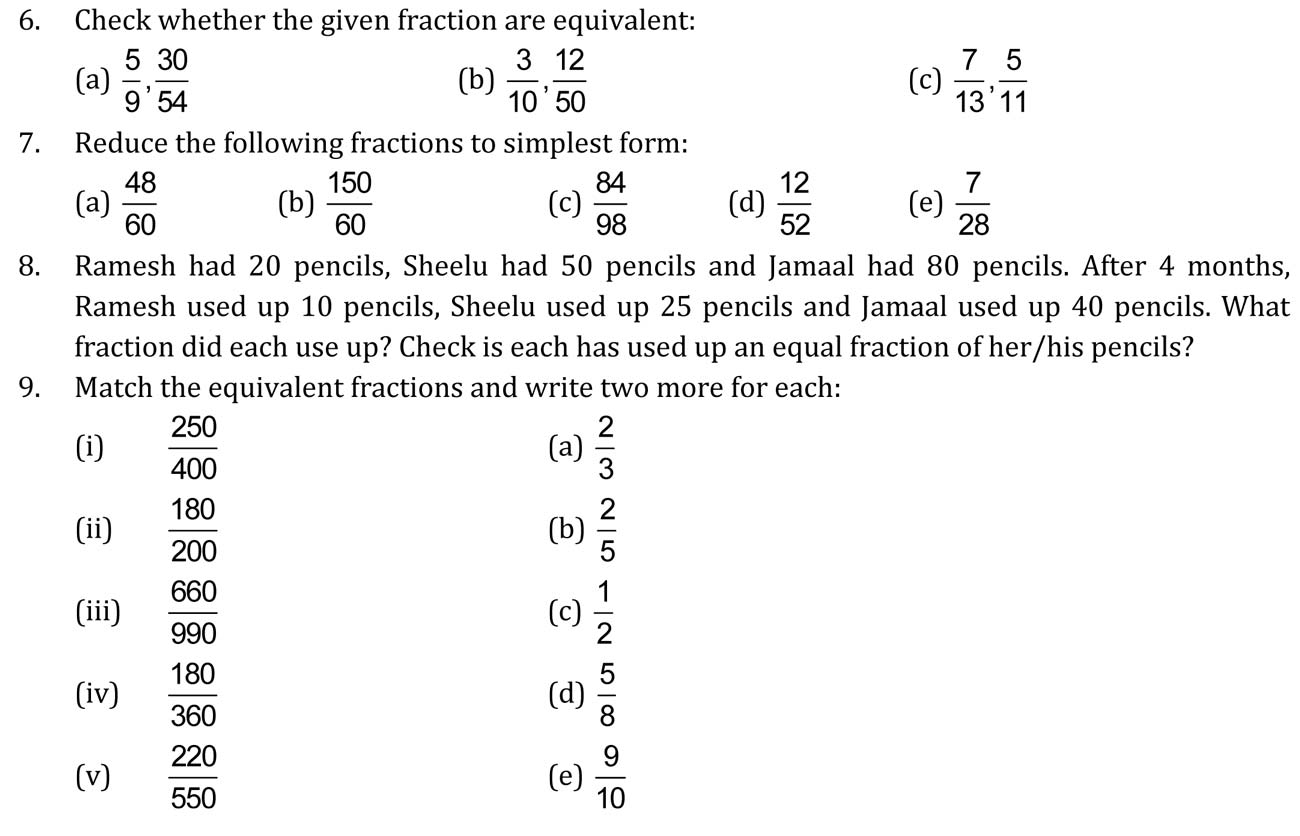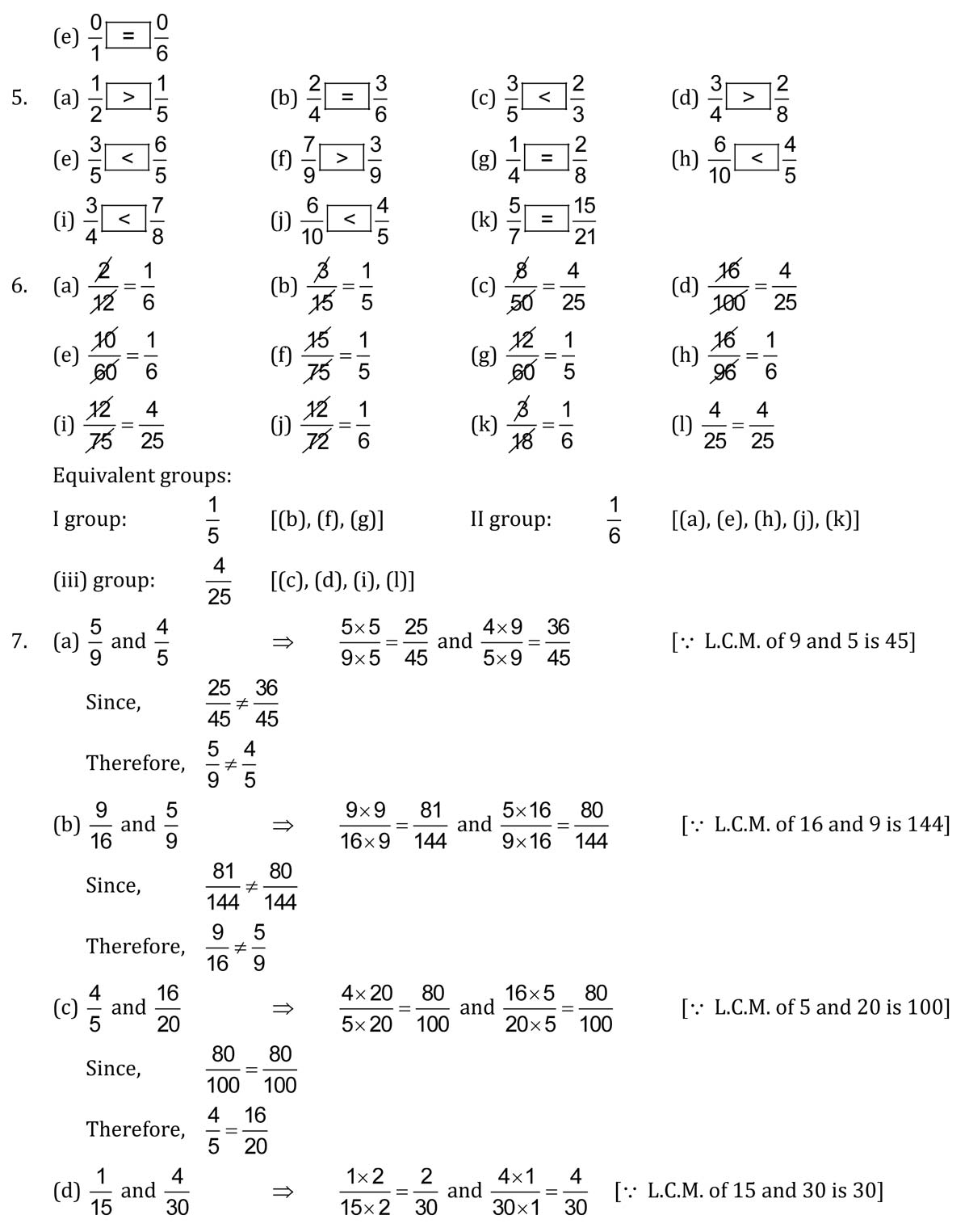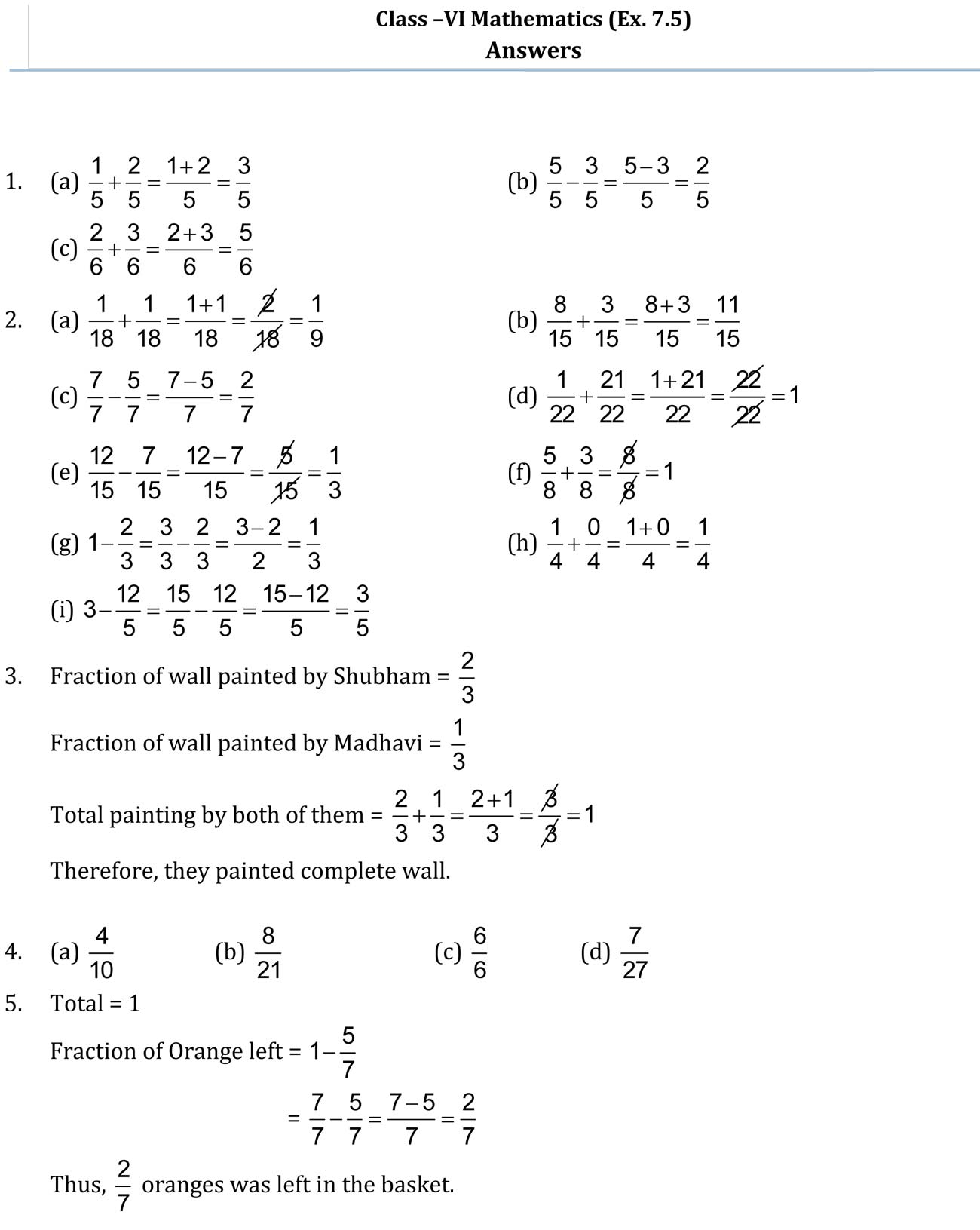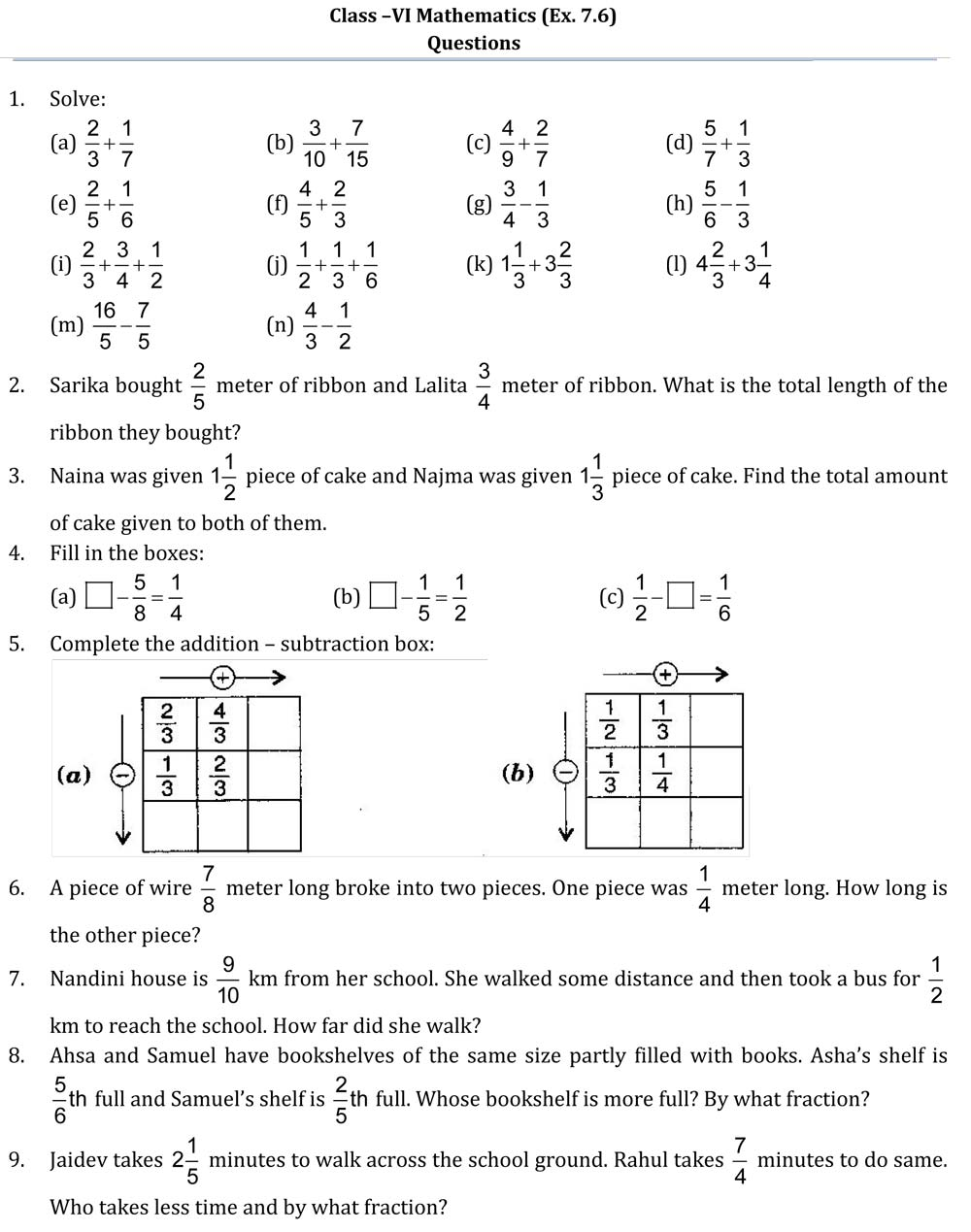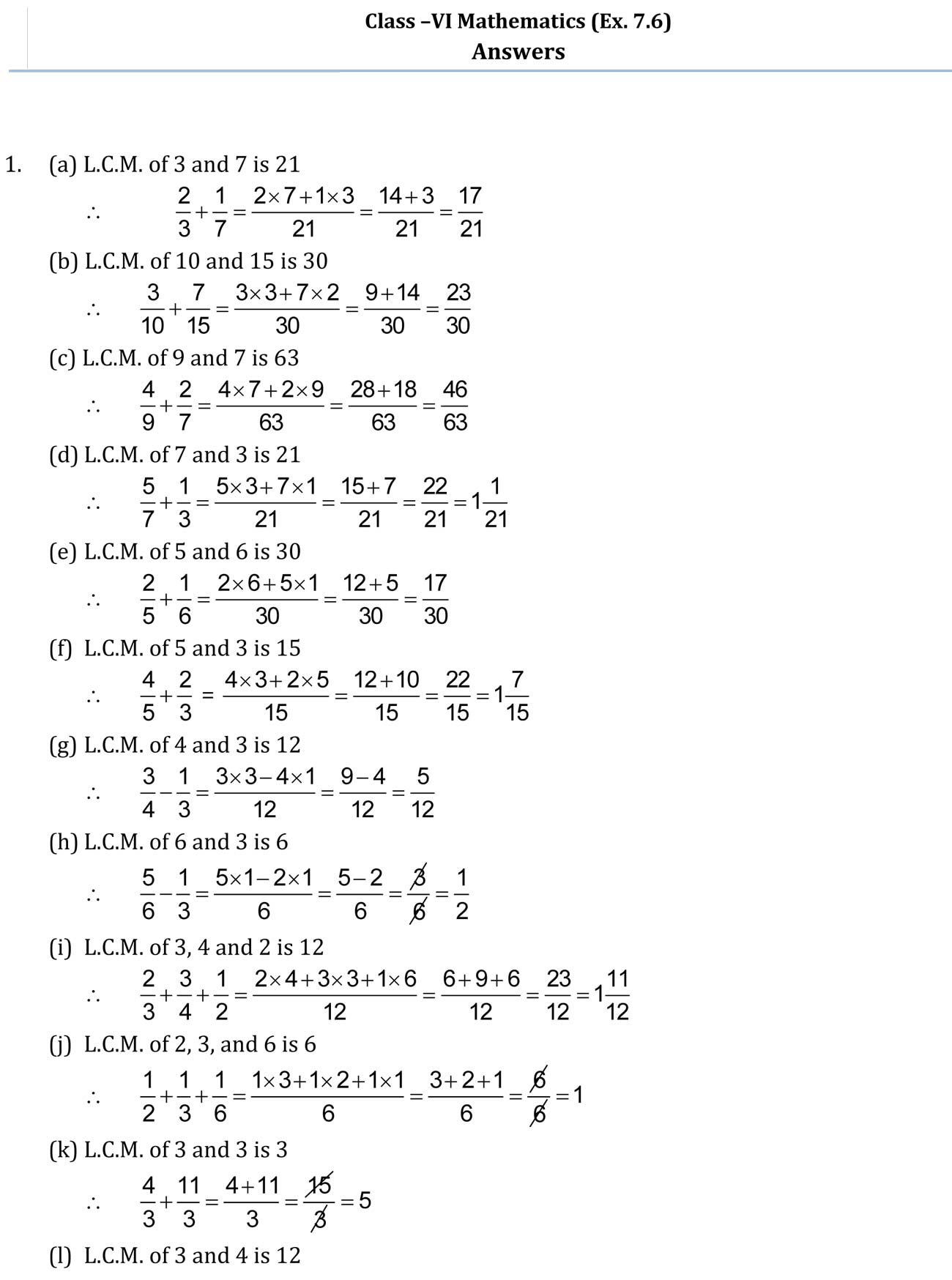# NCERT Solutions Class 6 Fractions

## Class 6 Maths Chapter 7 NCERT Solutions

### NCERT Solutions Class 6 Maths Chapter 7 PDF

Students studying in class 6 are already familiar with the term fractions, but in this chapter, they will be learning advanced topics related to fractions. They will learn how to represent a fraction on a number line, proper fractions. Proper fractions mean the numbers which are less than 1 like ¾, ⅚, 0/3 and they life left of 1st they are less than 1. To learn the concepts of fractions try to solve the exercise questions provided in the NCERT book. If you feel any you are stuck at any questions you can refer to NCERT solutions for class 6 chapter 7 fractions provided here in PDF format.